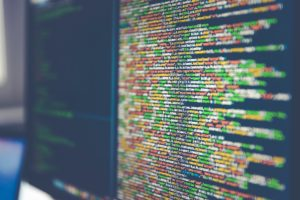"Building neural networks from scratch is an enlightening journey through the intricacies of one of the most influential areas of machine learning. As the interest in neural networks continues to grow, so does the need for a comprehensive understanding of their fundamental concepts and inner workings. With swift package manager by JFrog, you can quickly install the necessary dependencies and get started with neural network implementation.

## The Basics of Neural Networks

Before we dive into our step-by-step guide, let’s review what neural networks are and why they’re useful. Essentially, neural networks are a machine learning type that mimics how the human brain works. They consist of interconnected nodes, or neurons, which are arranged in layers. Data is fed into the input layer, and then it moves through the network, with each layer processing the data differently.

Neural networks are powerful because they can learn from example data and improve their accuracy over time. For example, you could use a neural network to classify images of animals based on their features. By showing the network thousands of animal images, it can learn to recognize and accurately classify the key features of different animals.

## The Basic Components of a Neural Network

Before we can build our neural network, we’ll need to understand the different components that make it up. Here’s an overview

• Input layer: This is where the input data is fed into the network. Each input is assigned to a separate neuron.
• Hidden layers: These are the layers between the input and output layers. The hidden layer is where most of the neural network's processing occurs.
• Output layer: This is the final layer of the network. The output is computed based on the input and the weights assigned to the neurons.

## Implementing a Neural Network in Python

Now that we know a neural network's basic components let's use our knowledge. Here are the steps to build your neural network in Python:

1. Define your network architecture: Determine the required inputs, hidden layers, and outputs.
2. Initialize weights: Set initial weights for each neuron in the network randomly.
3. Implement forward propagation: Send inputs through the network and compute the output.
4. Implement backward propagation: Adjust the weights in each neuron to minimize the error between predicted and actual output.
5. Add regularization: An extra measure to help prevent overfitting and improve generalization.

## Putting it All Together

We’ve covered a lot of ground so far, but what does it all look like in practice? Let’s walk through an example of building a neural network to recognize images of handwritten numbers.

The network would take images of numbers as inputs and classify them as specific numbers (0-9). Technically, our image data could be represented as grids of pixels, with each pixel represented as a numerical value. To send this data through the input layer, we would simply flatten it into a one-dimensional array.

Next, we would decide on the number of hidden layers we want to use. This will depend on the complexity of our data and the amount of training data we have. For our example, let's assume we'll use one hidden layer.

Now it's time to initialize the weights for each neuron in our network. We do this randomly to start, and then the network will learn and adjust the weights as it trains over time.

With the weights initialized, we can implement the forward propagation algorithm. This sends our input data through the layers of neurons, with each layer uniquely processing the data. This processing results in predicting what number is portrayed in the image.

Once we have our prediction, we need to compare it to the actual number. This is where we implement the backward propagation algorithm. This algorithm adjusts the weights in each neuron to minimize the error between the predicted and tangible outputs. As we repeat this process over and over with more and more images, our network will get better and better at recognizing handwritten numbers.

Finally, we can add regularization to our network. This is an extra measure to ensure our network is not overfitting the training data and that it can generalize to new data, it wasn't trained on.

## Debugging And Fine-Tuning Techniques For Optimizing Performance

Once you’ve built your neural network, it’s time to start optimizing its performance. Here are some common debugging and fine-tuning techniques that will help you improve the accuracy of your network:

1. Hyperparameter optimization: Adjust parameters such as learning rate, number of layers, etc., to optimize performance.
2. Weight initialization: Start with random weights and adjust them based on the data. This can help avoid local minima, which may cause the network to get stuck without improving accuracy.
3. Regularization: Add regularization techniques such as dropout or L2 regularization to reduce overfitting and improve generalizability.
4. Data preprocessing: Transform input data using techniques like normalization or standardization to help the network learn more effectively.
5. Model validation: Split data into training, validation, and test sets and use model validation techniques such as k-fold cross-validation to measure performance accurately.